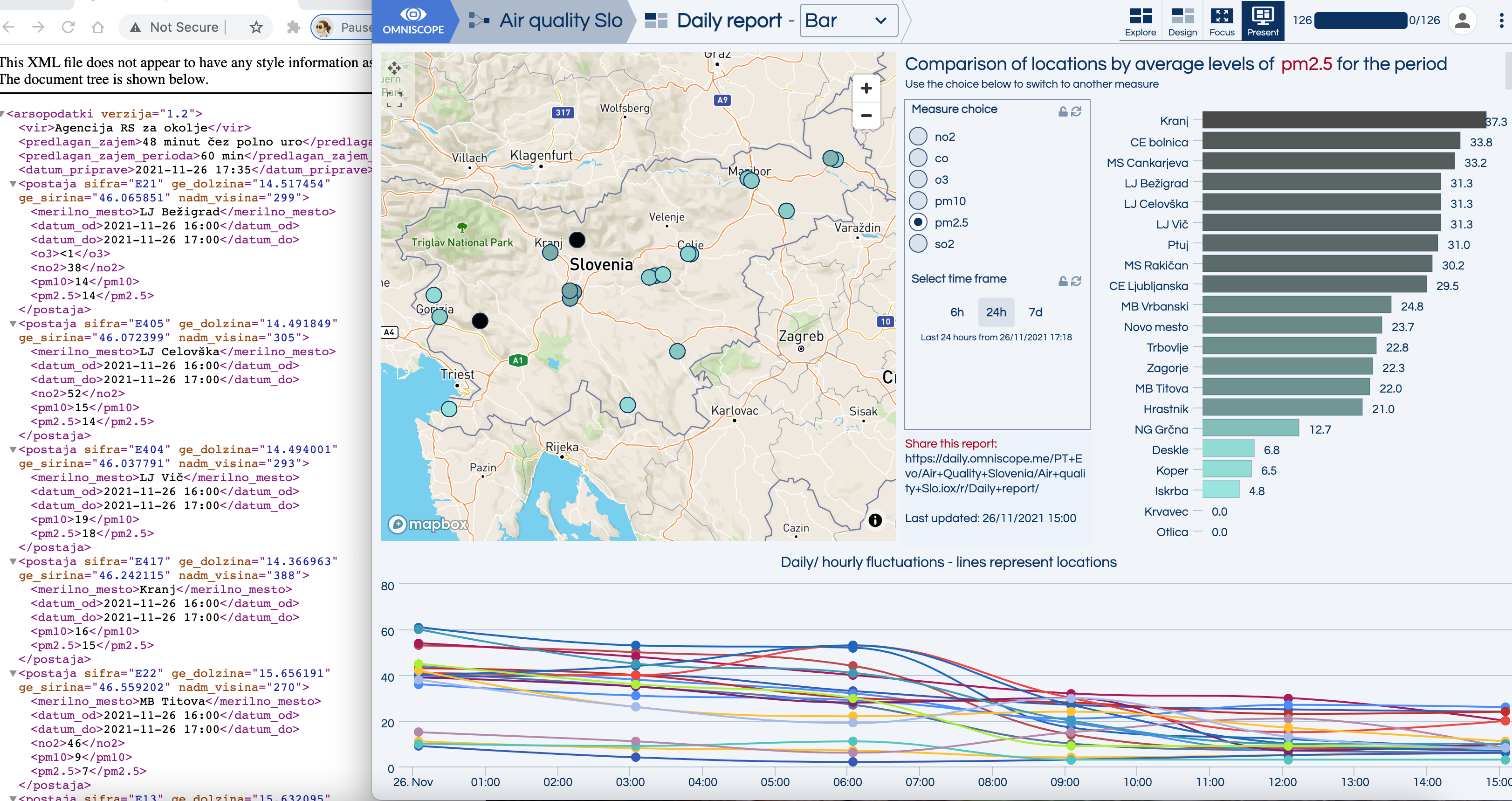## 1. 创建自动化数据采集工作流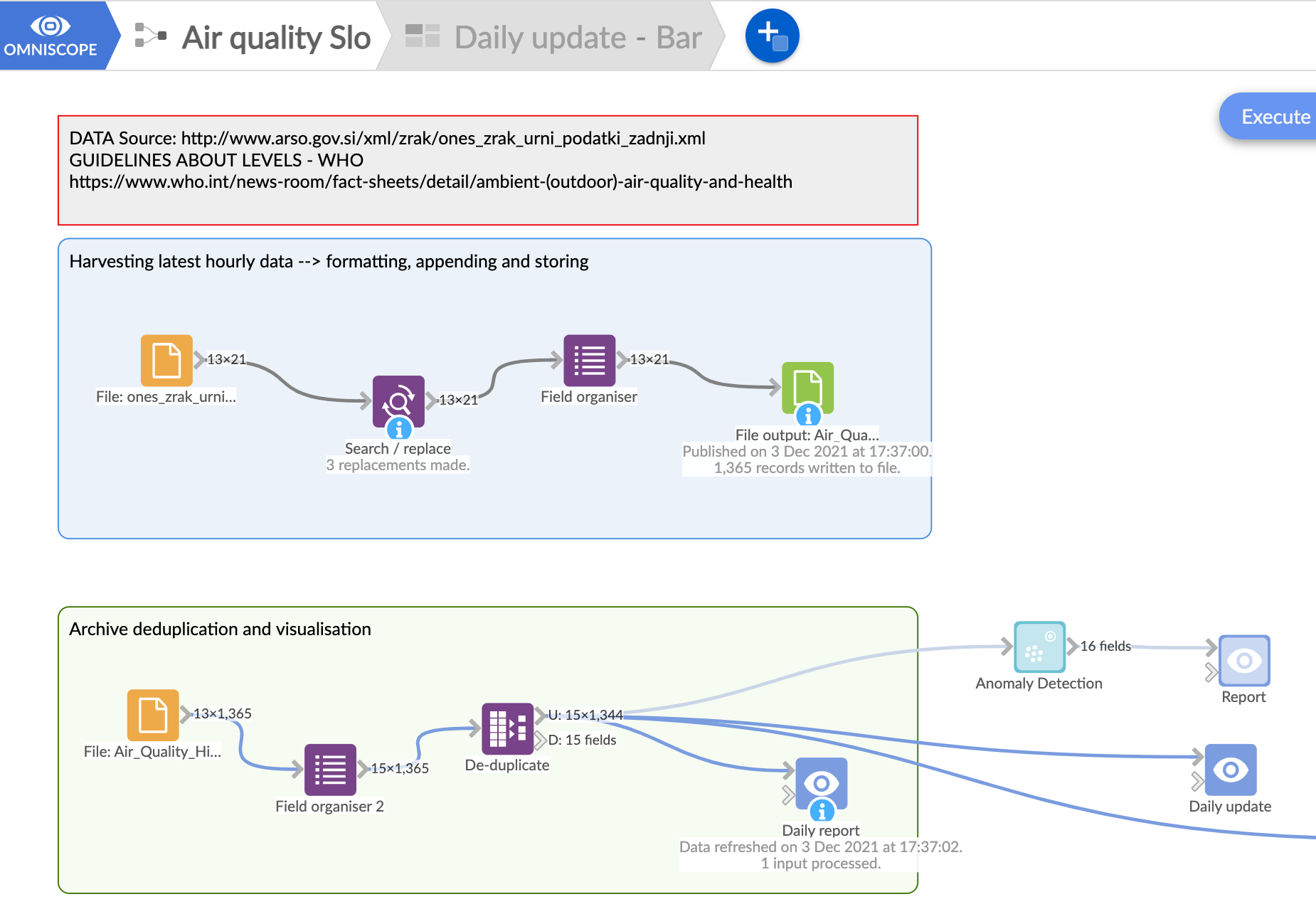## 2. 使用过滤器和 KPI 视图构建动态仪表板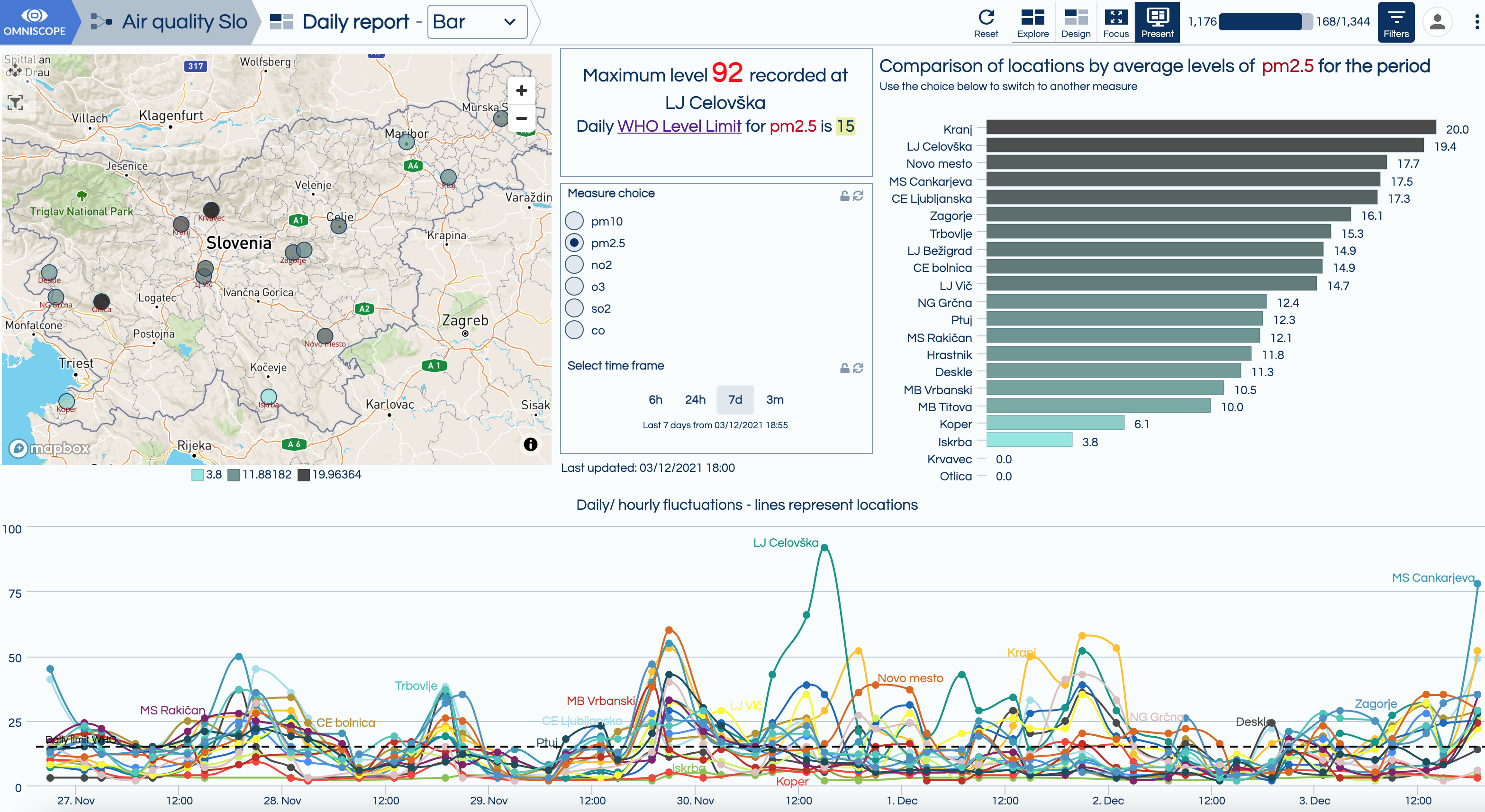IF(

[Measure choice]=”co”,[co],

[Measure choice]=”no2″,[no2],

[Measure choice]=”o3″,[o3],

[Measure choice]=”pm10″,[pm10],

[Measure choice]=”pm2.5″,[pm2.5],

[so2]

)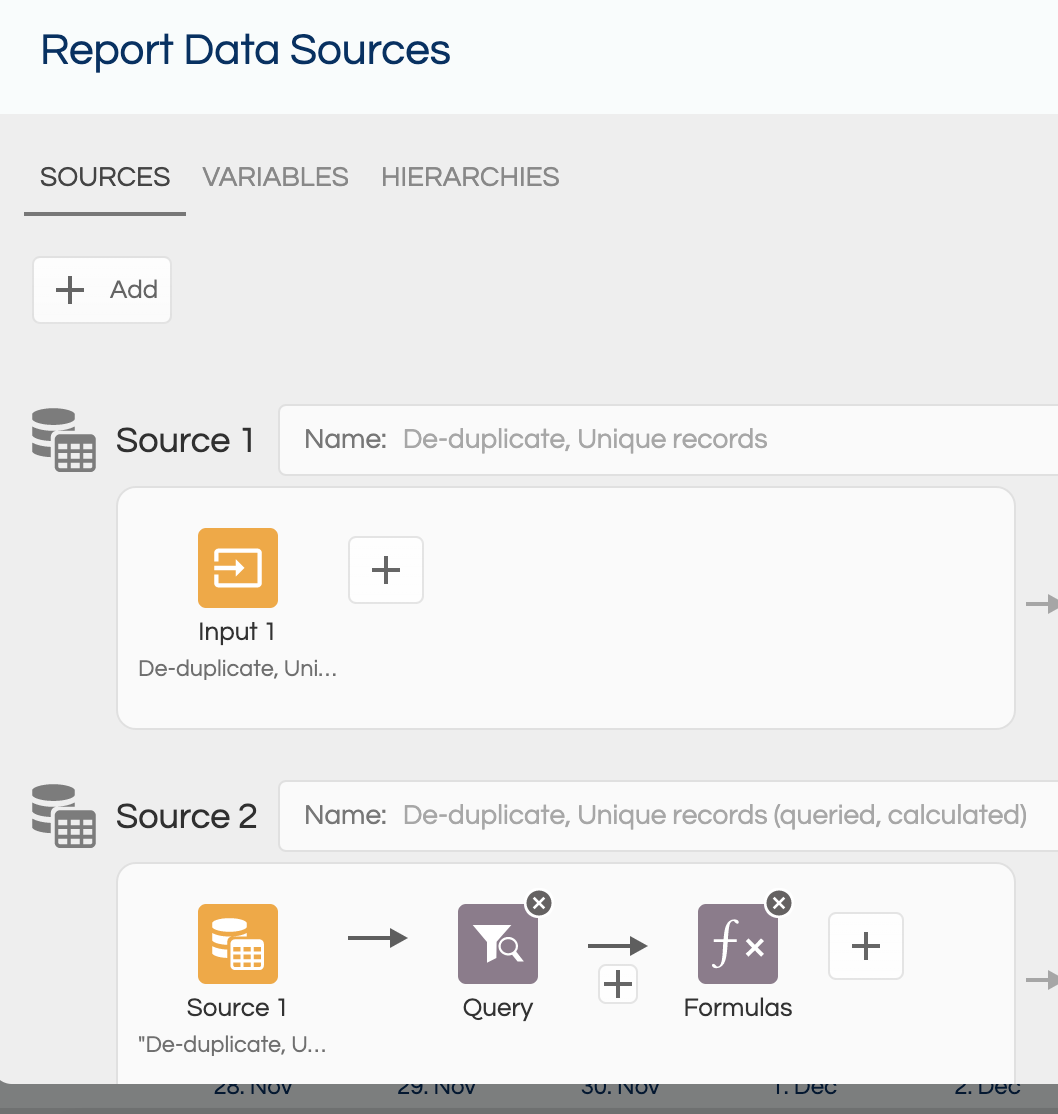### 如何构建交互式 KPI 视图IF(

SUBSET_MAX([Measure])=null,”no measurements”,

SUBSET_MAX([Measure])

)

SUBSET_UNIQUESLIST([merilno.mesto],

SUBSET([Measure],(SUBSET_MAX([Measure]))))

IF(

SUBSET_MAX([Measure])

IF([Measure choice]=”pm10″,45,

[Measure choice]=”pm2.5″,15,

[Measure choice]=”no2″,25,

[Measure choice]=”o3″,100,

[Measure choice]=”so2″,40,

[Measure choice]=”co”,35,

null)

0,”red”,”green”)

IF(

[Measure choice]=”pm10″,45,

[Measure choice]=”pm2.5″,15,

[Measure choice]=”no2″,25,

[Measure choice]=”o3″,100,

[Measure choice]=”so2″,40,

[Measure choice]=”co”,35,

null)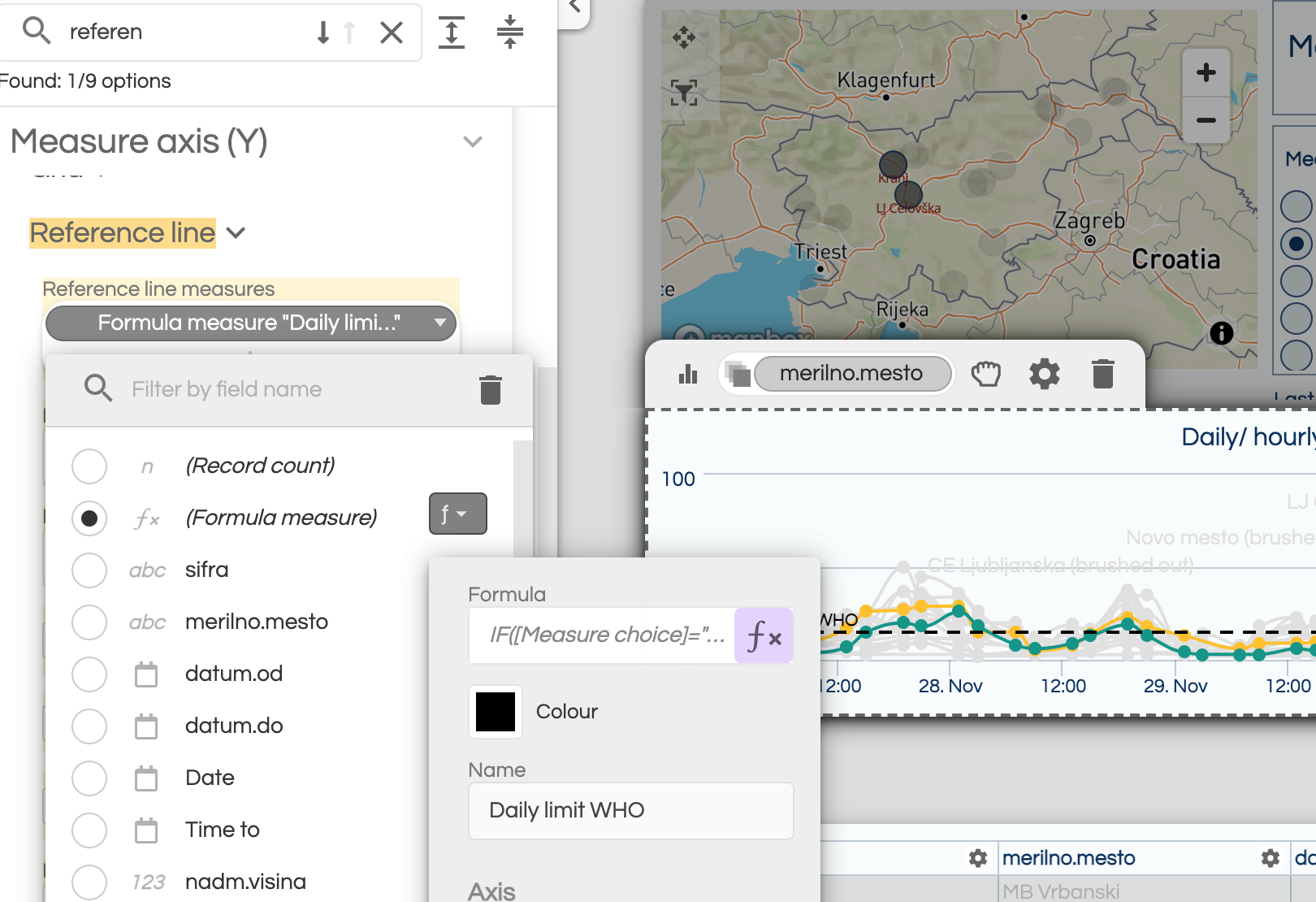## 3.样式和润色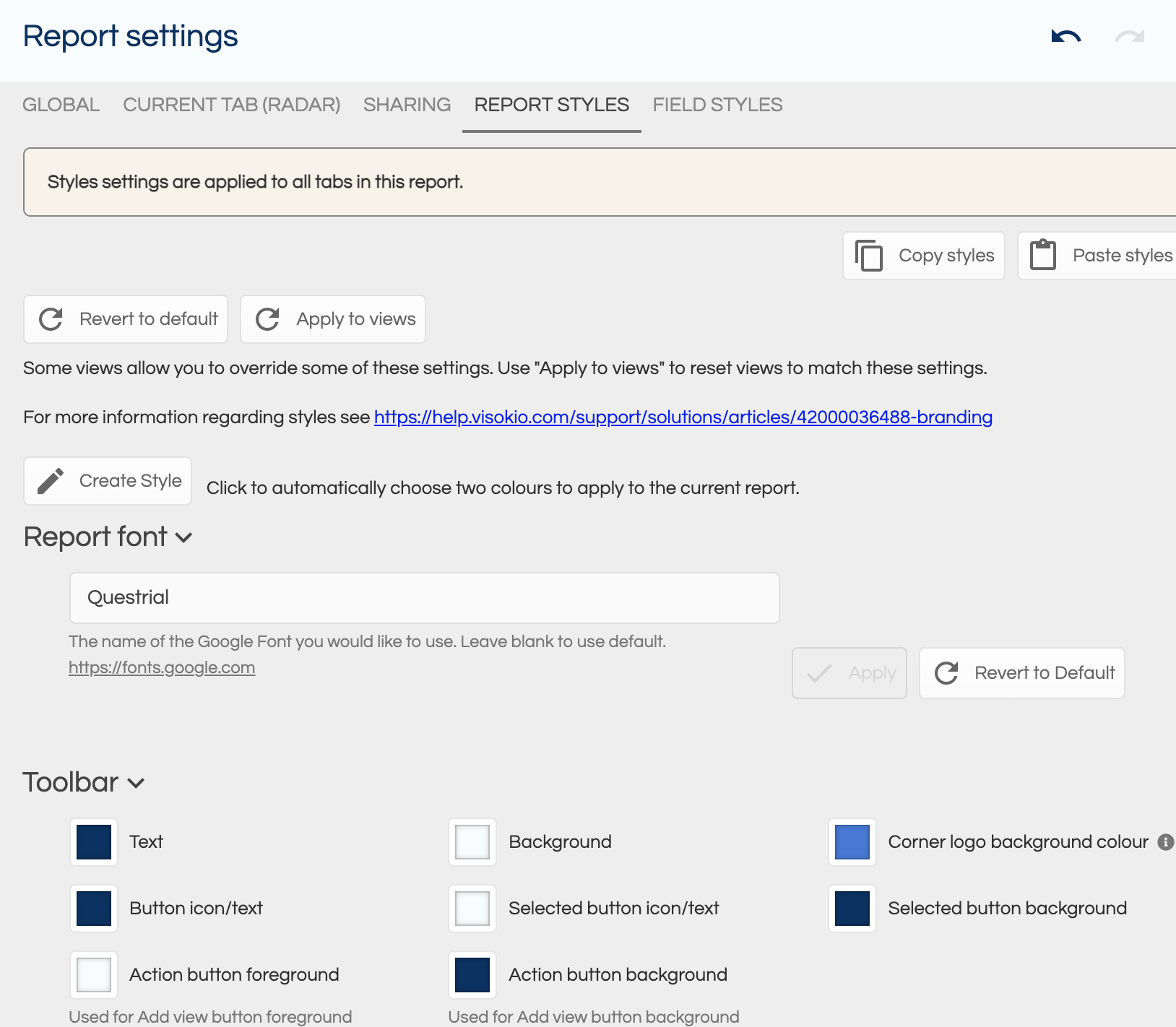• 选择默认数据颜色
• 单击“分配颜色”，对每个字段中的值使用随机分配
• 通过单击左侧边栏数据菜单中的数据值旁边的颜色方块，手动选择颜色